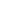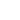AI

# TensorFlow还是Keras？深度学习框架选型指南

【编者按】到底该选择TensorFlow还是Keras，是深度学习初学者经常纠结的一个问题。数据科学家Aakash Nain比较了TensorFlow和Keras，希望有助于选择合适的框架。#### 快速开发原型

``````model = Sequential()
model.compile(optimizer='rmsprop',
loss='binary_crossentropy',
metrics=['accuracy'])

# 生成虚假数据
import numpy as np
data = np.random.random((1000, 100))
labels = np.random.randint(2, size=(1000, 1))

# 训练模型，迭代数据（每个batch包含32个样本）
model.fit(data, labels, epochs=10, batch_size=32)
``````

#### 没有人不喜欢Pythonic！！

Keras的开发设计注重用户友好，因而某种意义上它更加pythonic。模块化是Keras的另一个优雅的设计指导原则。Keras中的任何东西都可以表示为模块，用户可以根据需要将其进一步组合。

#### 功能性

``````# 创建图，等等。
init_op = tf.global_variables_initializer()

# 创建会话，以运行图中的操作。
sess = tf.Session()

# 初始化变量（比如epoch计数器）
sess.run(init_op)

# 开始输入入队线程。
coord = tf.train.Coordinator()
threads = tf.train.start_queue_runners(sess=sess, coord=coord)

try:
while not coord.should_stop():
# 运行训练步骤，等等
sess.run(train_op)

except tf.errors.OutOfRangeError:
print('训练完毕——达到epoch限制')

finally:
# 结束时，请求停止线程
coord.request_stop()

# 等待线程终止。
sess.close()
``````

TensorFlow有一个专门的调试器，为内部结构和正在运行的TensorFlow图的状态提供了可见性。从调试器获得的洞见可以加速调试训练和推断阶段的多种bug。TensorFlow调试器截屏（来源：TensorFlow文档）

#### 控制

``````step = tf.Variable(1, trainable=False, dtype=tf.int32)
``````

``````# 创建优化器

# 为一组变量计算梯度

# 你可以随意对梯度部分进行操作，比如，每项减1.
subtracted_grads_and_vars = [(gv - 1.0, gv) for gv in grads_and_vars]

# 请求优化器应用减一后的梯度。
``````

（以上代码样例取自 CS 20SI: TensorFlow for Deep Learning Research）

#### 结论（TL;DR）

`tf.contrib.keras` + `tf` = `你所需要的一切`

### 评论游客2017-11-28

2017-11-02

2017-10-25

2017-07-25

### 让平面变立体——特定类别3D网格重建学习发布需求联系客服